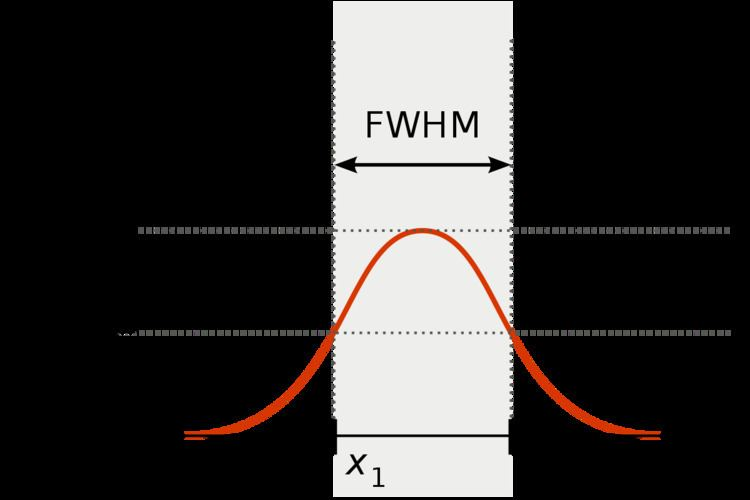# Full width at half maximum

Updated on
Edit
Like
CommentFull width at half maximum (FWHM) is an expression of the extent of a function given by the difference between the two extreme values of the independent variable at which the dependent variable is equal to half of its maximum value. In other words, it is the width of a spectrum curve measured between those points on the y-axis which are half the maximum amplitude.

Half width at half maximum (HWHM) is half of the FWHM.

FWHM is applied to such phenomena as the duration of pulse waveforms and the spectral width of sources used for optical communications and the resolution of spectrometers.

The term full duration at half maximum (FDHM) is preferred when the independent variable is time.

The convention of "width" meaning "half maximum" is also widely used in signal processing to define bandwidth as "width of frequency range where less than half the signal's power is attenuated", i.e., the power is at least half the maximum. In signal processing terms, this is at most −3 dB of attenuation, called "half power point".

If the considered function is the density of a normal distribution of the form

f ( x ) = 1 σ 2 π exp [ ( x x 0 ) 2 2 σ 2 ]

where σ is the standard deviation and x0 is the expected value, then the relationship between FWHM and the standard deviation is

F W H M = 2 2 ln 2 σ 2.355 σ .

The width does not depend on the expected value x0; it is invariant under translations.

In spectroscopy half the width at half maximum (here γ), HWHM, is in common use. For example, a Lorentzian/Cauchy distribution of height 1/πγ can be defined by

f ( x ) = 1 π γ [ 1 + ( x x 0 γ ) 2 ]  and  F W H M = 2 γ .

Another important distribution function, related to solitons in optics, is the hyperbolic secant:

f ( x ) = sech ( x X ) .

Any translating element was omitted, since it does not affect the FWHM. For this impulse we have:

F W H M = 2 arsech ( 1 2 ) X = 2 ln ( 2 + 3 ) X 2.634 X

where arsech is the inverse hyperbolic secant.

Full width at half maximum Wikipedia